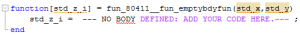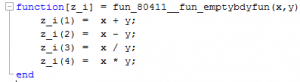# Use of Functions IV – Definition by Interface

Functions that are defined by interfaces in MOSAICmodeling can also be called “empty body functions”, because only inputs and outputs and no function bodies are specified inside these MOSAICmodeling functions. They can be used as placeholders in equation systems. The output and input variables must be defined via an interface whereas the mathematical expression doesn’t have to be specified at that point. In this way the equation system is independent from the functional correlation used to calculate the output variable. Similar to functions with defined bodies several variable inputs are possible while only one output variable is calculated. Both input and output variables can be scalars or vectors. Hence more than one functional dependence can be expressed by the use of one empty body function.

Problem description

In this exercise the use of functions defined by interfaces is practiced. An equation system with following mathematical operations will be created:$z_1 = x + y$              (1)$z_2 = x - y$              (2)$z_3 = \frac{x}{y}$                    (3)$z_4 = x \cdot y$                (4)$Z = \sum^{4}_{i=1} z_i$           (5)
.

First an empty body function is created in MOSAICmodeling which will be filled with the mathematical expressions from equation (1) to (2) in Matlab afterwards. The function’s output variable$z_{i}$ is a vector. In the simulation$y$ shall be determined iteratively for a given$x$ and$Z$.

Exercise

1. Create an interface similar to table (1). Use the notation 2951 (“Public_notation.mosnot”). The input variables are scalar while the output variable is a vector.

Variable Naming Dim In/Out$x$ Scalar In$y$ Scalar In$z_i$ Vector Out

2. Set up following equation:$Z = \sum^{4}_{i=1} z_i$

referring to equation (5) and name it “eq_sumOfZ.mosequ”. Use the notation 2951 “Public_notation.mosnot” once again.

3. Open the “Function” editor, enter a meaningful description and change the “Interface settings” to “Load Interface”. Select the interface that was created before. The notation from the interface is adopted. Save the function as “fun_emptyBdyFun.mosfun”.
4. Build an equation system “eqs_calcValofY.moseqs” with a short description
and the notation 2951 “Public_notation.mosnot”. It has to contain the equation “eq_sumOfZ.mosequ” and the function “fun_emptyBdyFun.mosfun”. Use the same variable namings from the interface as applied namings for the function calls when editing the function calls.
5. Go to “Simulation”, load the equation system, set the maximum value in the “indexing” section to 4 and open the “Specification” tab. The degree of freedom should be 2. Select$x$ and$Z$ as design variables and set the value of$x$ to 4, and the value of$Z$ to 18. The initial value of$y$ can be 5.
6. Next open the “Evaluation” tab, change the predefined “Language Specification” to “MATLAB NLE” and click on “Generate Code”. Change to the tab “View Code”, press the button “Export Code” and open a convenient output path, where a simulation folder containing the m-file is added to.
7. Save the code as a function “solveEquationSystem.m” and open it in the Matlab editor.
8. Search for the lines:The number behind “fun_” equals the function ID in MOSAICmodeling. It differs from the one presented here.
9. Replace the lines from figure (1) by filling it with equation (1) to (4):Save the changes.
10. Run the program, what is the result of y ? (Its value is displayed in the command window)